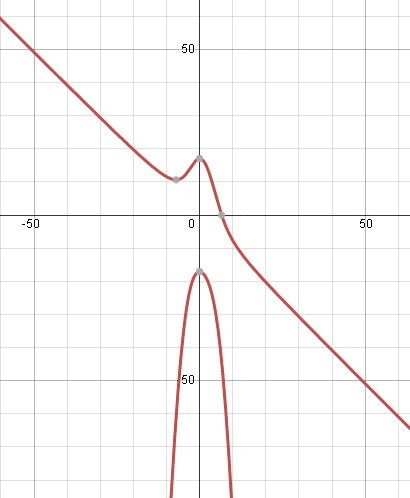Search IntMath
Close

450+ Math Lessons written by Math Professors and Teachers

5 Million+ Students Helped Each Year

1200+ Articles Written by Math Educators and Enthusiasts

Simplifying and Teaching Math for Over 23 Years

# How to differentiate? [Solved!]

### My question

Given: x^3+x^2y+y^2 = 292
Find: dy/dx, where : x=600, y= 200

### Relevant page

7c. Given dy/dx, find y = f(x)

### What I've done so far

Watched the videos and read some of the pages. But I'm lost

X

Given: x^3+x^2y+y^2 = 292
Find: dy/dx, where : x=600, y= 200
Relevant page

<a href="/integration/integration-mini-lecture-find-y-given-dy-dx.php">7c. Given dy/dx, find y = f(x)</a>

What I've done so far

Watched the videos and read some of the pages. But I'm lost

## Re: How to differentiate?

Hi Oscar

There are examples similar to your question on this Implicit Differentiation page:

8. Differentiation of Implicit Functions

Is that enough to get you started?

Post your solution so we can see how you went. I encourage you to use the math entry system to make your math easier to read.

X

Hi Oscar

There are examples similar to your question on this Implicit Differentiation page:

<a href="/differentiation/8-derivative-implicit-function.php">8. Differentiation of Implicit Functions</a>

Is that enough to get you started?

Post your solution so we can see how you went. I encourage you to use the math entry system to make your math easier to read.

## Re: How to differentiate?

Yah - I can see it's like your examples.

x^3+x^2y+y^2 = 292

3x^2 + x^2dy/dx + y(2x) + 2ydy/dx = 0

3x^2 + 2xy + (x^2 + 2y)dy/dx = 0

dy/dx = (3x^2 + 2xy)/(x^2 + 2y)

Now substituting x=600 and y=200

dy/dx = (3(600)^2 + 2(600)(200))/((600)^2 + 2(200))

=3.66

X

Yah - I can see it's like your examples.

x^3+x^2y+y^2 = 292

3x^2 + x^2dy/dx + y(2x) + 2ydy/dx = 0

3x^2 + 2xy + (x^2  + 2y)dy/dx = 0

dy/dx = (3x^2 + 2xy)/(x^2  + 2y)

Now substituting x=600 and y=200

dy/dx = (3(600)^2 + 2(600)(200))/((600)^2  + 2(200))

=3.66

## Re: How to differentiate?

You almost got it right - your dy/dx is missing a negative...

X

You almost got it right - your dy/dx is missing a negative...

## Re: How to differentiate?

Doh

Should have been:

dy/dx = -(3x^2 + 2xy)/(x^2 + 2y)

dy/dx = -(3(600)^2 + 2(600)(200))/((600)^2 + 2(200))

=-3.66

Thanks a lot.

X

Doh

Should have been:

dy/dx = -(3x^2 + 2xy)/(x^2  + 2y)

dy/dx = -(3(600)^2 + 2(600)(200))/((600)^2  + 2(200))

=-3.66

Thanks a lot.

## Re: How to differentiate?

For interest just now, I graphed your implicit function and noticed a problem.The graph doesn't even go through the point (600, 200)!

This is a case where we can calculate an answer, but it doesn't have any meaning.

As you can see, the graph as x gets very big (and goes off in the negative direction) has slope very close to -1.

When we substitute x=200 into the original equation, we get y^2+40000y+7999708 = 0 and this has solutions y=-39799 or y=-201.0.

The slope is steeply negative for the first one, and very close to -1 for the second.

Graphs tell us a lot about what is going on!

X

For interest just now, I graphed your implicit function and noticed a problem.

<img src="/forum/uploads/imf-4548-x3x2yy2is292-graph.png" width="410" height="498" alt="How to differentiate?" />

The graph doesn't even go through the point (600, 200)!

This is a case where we can calculate an answer, but it doesn't have any meaning.

As you can see, the graph as x gets very big (and goes off in the negative direction) has slope very close to -1.

When we substitute x=200 into the original equation, we get y^2+40000y+7999708 = 0 and this has solutions y=-39799 or y=-201.0.

The slope is steeply negative for the first one, and very close to -1 for the second.

Graphs tell us a lot about what is going on!

## Re: How to differentiate?

Thanks! I'll tell my teacher :-)

X

Thanks! I'll tell my teacher :-)